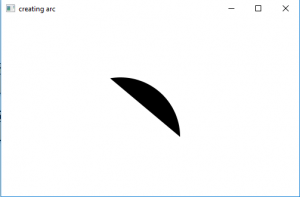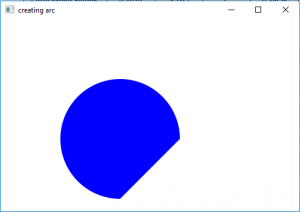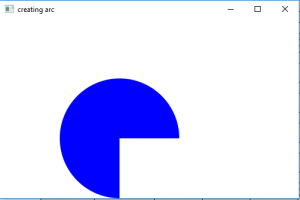Related Articles
JavaFX | Arc with examples
• Last Updated : 13 May, 2019

Arc class is a part of JavaxFX. Arc class creates an arc on some given values specified, such as center of the arc, the start angle, the extent of arc(length), and radius. Arc class extends Shape class.

Constructor for the class are

1. Arc(): creates an empty instance of arc class
2. Arc(double centerX, double centerY, double radiusX, double radiusY, double startAngle, double length): creates an arc with given coordinates and values

commonly used methods

Method Explanation
getCenterX() returns the x coordinate of the center of arc
getCenterY() returns the y coordinate of the center of arc
getStartAngle() returns the start angle of the arc
getType() Gets the value of the property type.
setCenterX(double v) sets the x coordinate of the center of arc
setCenterY(double v) sets the y coordinate of the center of arc
setLength(double v) sets the length of the arc
setStartAngle(double v) sets the start angle of the arc
setType(ArcType v) sets the arc type of the arc

The following programs will illustrate the use of the Arc class

Java program to create a arc
This program creates a Arc indicated by the name arc( center, radius, start angle and arc length is passed as arguments). The arc will be created inside a scene, which in turn will be hosted inside a stage. The function setTitle() is used to provide title to the stage. Then a Group is created, and the arc is attached.The group is attached to the scene. Finally, the show() method is called to display the final results.

 `// Java program to create a arc ` `import` `javafx.application.Application; ` `import` `javafx.scene.Scene; ` `import` `javafx.scene.shape.DrawMode; ` `import` `javafx.scene.layout.*; ` `import` `javafx.event.ActionEvent; ` `import` `javafx.scene.shape.Arc; ` `import` `javafx.scene.control.*; ` `import` `javafx.stage.Stage; ` `import` `javafx.scene.Group; ` `public` `class` `arc_0 ``extends` `Application { ` ` `  `    ``// launch the application ` `    ``public` `void` `start(Stage stage) ` `    ``{ ` `        ``// set title for the stage ` `        ``stage.setTitle(``"creating arc"``); ` ` `  `        ``// create a arc ` `        ``Arc arc = ``new` `Arc(``100``.0f, ``100``.0f, ``100``.0f, ``100``.0f, ``0``.0f, ``100``.0f); ` ` `  `        ``// create a Group ` `        ``Group group = ``new` `Group(arc); ` ` `  `        ``// translate the arc to a position ` `        ``arc.setTranslateX(``100``); ` `        ``arc.setTranslateY(``100``); ` ` `  `        ``// create a scene ` `        ``Scene scene = ``new` `Scene(group, ``500``, ``300``); ` ` `  `        ``// set the scene ` `        ``stage.setScene(scene); ` ` `  `        ``stage.show(); ` `    ``} ` ` `  `    ``public` `static` `void` `main(String args[]) ` `    ``{ ` `        ``// launch the application ` `        ``launch(args); ` `    ``} ` `} `

Output:Java program to create a arc and set fill for the arc

This program creates a Arc indicated by the name arc( center, radius, start angle and arc length are set using setCenterX(), setCenterY(), setRadiusX(), setRadiusY(), setLength()). The arc will be created inside a scene, which in turn will be hosted inside a stage. The function setTitle() is used to provide title to the stage. Then a Group is created, and the arc is attached.The group is attached to the scene. Finally, the show() method is called to display the final results. The function setFill() is used to set fill for the arc.

 `// Java program to create a arc ` `// and set fill for the arc ` `import` `javafx.application.Application; ` `import` `javafx.scene.Scene; ` `import` `javafx.scene.shape.DrawMode; ` `import` `javafx.scene.layout.*; ` `import` `javafx.event.ActionEvent; ` `import` `javafx.scene.shape.Arc; ` `import` `javafx.scene.control.*; ` `import` `javafx.stage.Stage; ` `import` `javafx.scene.Group; ` `import` `javafx.scene.shape.ArcType; ` `import` `javafx.scene.paint.Color; ` `public` `class` `arc_1 ``extends` `Application { ` ` `  `    ``// launch the application ` `    ``public` `void` `start(Stage stage) ` `    ``{ ` `        ``// set title for the stage ` `        ``stage.setTitle(``"creating arc"``); ` ` `  `        ``// create a arc ` `        ``Arc arc = ``new` `Arc(); ` ` `  `        ``// set center ` `        ``arc.setCenterX(``100``.0f); ` `        ``arc.setCenterY(``100``.0f); ` ` `  `        ``// set radius ` `        ``arc.setRadiusX(``100``.0f); ` `        ``arc.setRadiusY(``100``.0f); ` ` `  `        ``// set start angle and length ` `        ``arc.setStartAngle(``0``.0f); ` `        ``arc.setLength(``270``.0f); ` ` `  `        ``// create a Group ` `        ``Group group = ``new` `Group(arc); ` ` `  `        ``// translate the arc to a position ` `        ``arc.setTranslateX(``100``); ` `        ``arc.setTranslateY(``100``); ` ` `  `        ``// set fill for the arc ` `        ``arc.setFill(Color.BLUE); ` ` `  `        ``// create a scene ` `        ``Scene scene = ``new` `Scene(group, ``500``, ``300``); ` ` `  `        ``// set the scene ` `        ``stage.setScene(scene); ` ` `  `        ``stage.show(); ` `    ``} ` ` `  `    ``public` `static` `void` `main(String args[]) ` `    ``{ ` `        ``// launch the application ` `        ``launch(args); ` `    ``} ` `} `

Output:Java Program to create a arc and specify its fill and arc type

This program creates a Arc indicated by the name arc( center, radius, start angle and arc length are set using setCenterX(), setCenterY(), setRadiusX(), setRadiusY(), setLength()). The arc will be created inside a scene, which in turn will be hosted inside a stage. The function setTitle() is used to provide title to the stage. Then a Group is created, and the arc is attached.The group is attached to the scene. Finally, the show() method is called to display the final results. The function setFill() is used to set fill for the arc and function setArcType() is used to set ArcType of the arc.

 `// Java Program to create a arc and specify its fill and arc type ` `import` `javafx.application.Application; ` `import` `javafx.scene.Scene; ` `import` `javafx.scene.shape.DrawMode; ` `import` `javafx.scene.layout.*; ` `import` `javafx.event.ActionEvent; ` `import` `javafx.scene.shape.Arc; ` `import` `javafx.scene.control.*; ` `import` `javafx.stage.Stage; ` `import` `javafx.scene.Group; ` `import` `javafx.scene.shape.ArcType; ` `import` `javafx.scene.paint.Color; ` `public` `class` `arc_2 ``extends` `Application { ` ` `  `    ``// launch the application ` `    ``public` `void` `start(Stage stage) ` `    ``{ ` `        ``// set title for the stage ` `        ``stage.setTitle(``"creating arc"``); ` ` `  `        ``// create a arc ` `        ``Arc arc = ``new` `Arc(); ` ` `  `        ``// set center ` `        ``arc.setCenterX(``100``.0f); ` `        ``arc.setCenterY(``100``.0f); ` ` `  `        ``// set radius ` `        ``arc.setRadiusX(``100``.0f); ` `        ``arc.setRadiusY(``100``.0f); ` ` `  `        ``// set start angle and length ` `        ``arc.setStartAngle(``0``.0f); ` `        ``arc.setLength(``270``.0f); ` ` `  `        ``// create a Group ` `        ``Group group = ``new` `Group(arc); ` ` `  `        ``// translate the arc to a position ` `        ``arc.setTranslateX(``100``); ` `        ``arc.setTranslateY(``100``); ` ` `  `        ``// set fill for the arc ` `        ``arc.setFill(Color.BLUE); ` ` `  `        ``// set Type of Arc ` `        ``arc.setType(ArcType.ROUND); ` ` `  `        ``// create a scene ` `        ``Scene scene = ``new` `Scene(group, ``500``, ``300``); ` ` `  `        ``// set the scene ` `        ``stage.setScene(scene); ` ` `  `        ``stage.show(); ` `    ``} ` ` `  `    ``public` `static` `void` `main(String args[]) ` `    ``{ ` `        ``// launch the application ` `        ``launch(args); ` `    ``} ` `} `

Output:Note :The above program might not run in an online IDE please use an offline compiler
Reference
https://docs.oracle.com/javase/8/javafx/api/javafx/scene/shape/Arc.html

My Personal Notes arrow_drop_up
Recommended Articles
Page :